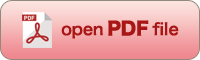[OPTICAL REVIEW Vol. 7, No. 2 (2000) 132-137]

An Acceleration Algorithm for Image Reconstruction Based on Continuous-Discrete Mapping Model

Taiga YAMAYA, Takashi OBI, Masahiro YAMAGUCHI and Nagaaki OHYAMA*

Imaging Science and Engineering Laboratory, Tokyo Institute of Technology, 4259, Nagatsuta, Midori-ku, Yokohama, 226-8503 Japan

(Received September 30, 1999; Accepted February 1, 2000)In image reconstruction for X-ray computed tomography, images reconstructed by filtered backprojection (FBP) include systematic errors because the FBP method does not take into account some of the properties of the actual imaging system such as the divergence of X-ray beam. One solution to this problem is to use algebraic reconstruction methods, such as generalized analytic reconstruction from discrete samples and natural pixel decomposition. However, in the process of reconstruction using these methods, it is necessary to solve the linear algebraic equations which have a large coefficient matrix. In this paper, we propose a method to accelerate the iteration solving these equations by preconditioning the coefficient matrix using a polynomial function. The results of the computer simulations show the effectiveness of the proposed method.

Key words : computed tomography, GARDS, NPD, continuous-discrete mapping model, conjugate gradient algorithm, preconditioning, acceleration, mass fractal, surface fractal, combined fractal, self-similarity, power law, fractal dimension

yama@isl.titech.ac.jp